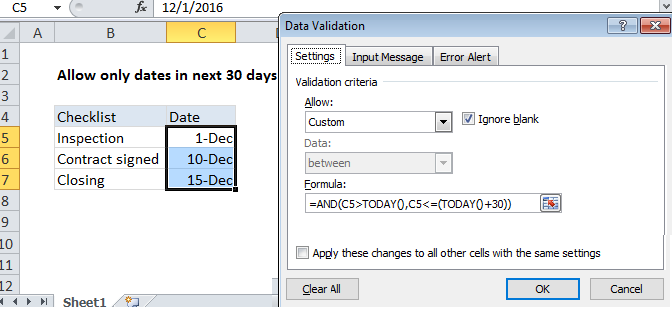## Excel Office

Excel How Tos, Tutorials, Tips & Tricks, Shortcuts

# Excel Data validation date in next 30 days

Using the example below, this tutorial shows how to create Data validation date in next 30 days in Excel.

## Formula

`=AND(A1>TODAY(),A1<=(TODAY()+days))`

##Explanation

Note: Excel has several built-in data validation rules for dates. This page explains how to create a your own validation rule based on a custom formula when you want more control and flexibility.

To allow only a date in the next 30 days, you can use data validation with a custom formula based on the AND, and TODAY functions.

Also See:   Highlight dates between in Excel

In the example shown, the data validation applied to C5:C7 is:

`=AND(C5>TODAY(),C5<=(TODAY()+30))`

### How this formula works

Data validation rules are triggered when a user adds or changes a cell value.

The TODAY function returns today’s date (recalculated an on on-going basis). The AND function takes multiple logical expressions and returns TRUE only when all expressions return TRUE. In this case, we need to test two conditions:

Also See:   Get first Monday before any date in Excel

The first condition checks that the input is greater than today:

`C5>TODAY()`

The second condition checks that the input is less than today + 30:

`C5<=(TODAY()+30)`

(Dates are just serial numbers in Excel, so we can simply add 30).

If both logical expressions return TRUE, the AND function returns TRUE and validation succeeds. If either expressions returns FALSE, data validation fails.

Also See:   TODAY function: Description, Usage, Syntax, Examples and Explanation

Note: Cell references in data validation formulas are relative to the upper left cell in the range selected when the validation rule is defined, in this case C5.